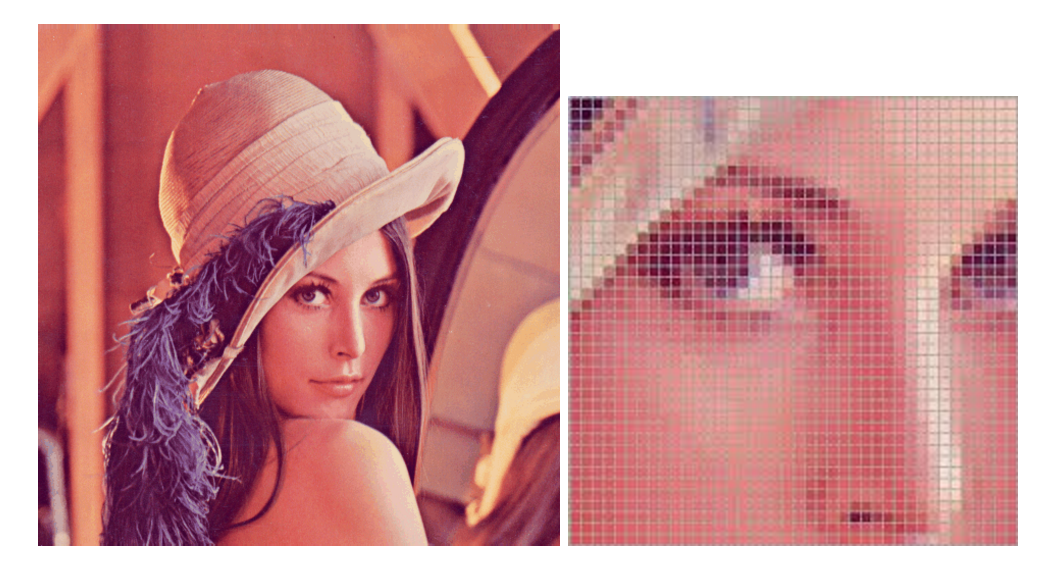## What is an Image?

In this section, we learn what is an image and what does it consists of.

### Basics

Below is the image of Lena which we use often in image processing. New algorithms are experimented on this image as it consists of diverse chunks like- curly hair, plain background, human face, etc.,Fig. (a) The image of lena, (b) Image of lena zoomed in

When you zoom very closely into the image, you will start to realize that the image is made up of discrete squares as shown in Fig. (b). Each of the discrete square have their own color. These discrete sqares are called picture elements, or in short pixels.

Every image is created similarly by a two dimensional array of discrete square which have specific colors. These small discrete squares (or blocks) come together to form a bigger image. The image in Fig. (a) has a high resolution (512x512) and hence you are unable to see the discrete square with your eye directly. There are so many small blocks because of which our eye renders the image to be continous.

### Image Resolution

The resolution of the image is the number of blocks in each of the directions in an image. It is represented by m x n when m is the number of pixels in x direction and n is the number of pixels in y direction.

For example, when I say the resolution of the image in Fig. (a) is 512x512, I mean that the number of blocks in x direction for the image is 512 and the number of blocks in y direction for the image is 512. That is a total of 262,144 pixels.

Let me give you another example, maybe a more intuitive one. When your phone manufacturer says that the resolution of the camera is 5 mega pixels (5 MP), what he means to say is that there will be a total of 5,000,000 pixels on the image that you take. So, the resolutions in x and y directions could be 2500 and 2000. So, the resolution of that image is 2500 x 2000.